# KVPY-SA 2018 Maths Paper with Solutions

Students can find the KVPY-SA 2018 Maths paper solutions on this page. These step by step solutions are prepared by specialized experts at BYJU’S. These solutions are given in a simple format so that students can easily understand the problems. The PDF format of the solutions is also available for offline practise. Revising these solutions will help students to be familiar with the type of questions asked for the KVPY exam.

### KVPY SA 2018 - Maths

Question 1: The number of pairs (a, b) of positive real numbers satisfying a4 + b4< 1 and a2 + b2> 1 is

1. a. 0
2. b. 1
3. c. 2
4. d. more than 2

Solution:

Let a2 = p and b2 = q here p > 0 & q > 0

Now, given in equation are,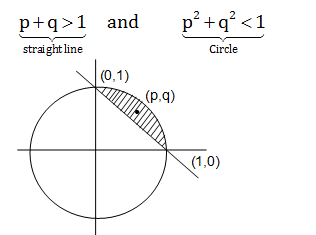Point (p, q) lies above x + y = 1 and (p, q) lies inside circle x2 + y2 = 1 {using concept of position of point}.

From common shaded region we can say (p, q) lies in shaded region and number of points in shaded region are infinite. So number of pairs (a, b) are also infinite.

Question 2: The number of real roots of the polynomial equation x4–x2 +2x–1 = 0 is

1. a. 0
2. b. 2
3. c. 3
4. d. 4

Solution:

⇒ x4–x2+2x –1 = 0 (given)

⇒ x4 – [x2–2x+1] = 0 { since A2–2AB+B2 = (A – B)2; A = x, B = 1}

⇒ x4 – (x –1)2 = 0

⇒ (x2–x+1) (x2 +x–1) = 0 {since a2-b2 = (a – b) (a + b); a = x2, b = x–1}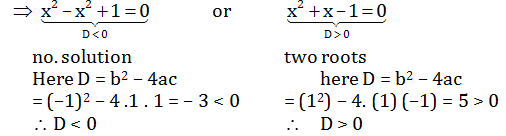D = Discriminant

b = coefficient of x

c = constant term

Question 3: Suppose the sum of the first m terms of arithmetic progression is n and the sum of its first n terms is m, where m ≠ n. Then the sum of the first ( m + n) terms of the arithmetic progression is

1. a. 1–mm
2. b. mn–5
3. c. –(m+n)
4. d. m+n

Solution:

Sm = (m/2)(2a+(m-1)d) = n …(1)

Since Sn = (n/2)(2a+(n-1)d

Sn = sum of n terms;

n = number of terms

a = first term;

d = common difference

Sn = (n/2)(2a+(n-1)d = m …(2)

From equation(1) – (2)

= ½ [2a(m–n) +d(m2 – m – n2 + n) = n – m

= ½ [2a(m–n) + d(m–n)(m +n–1)] = n – m

= ½ [2a + d (m + n – 1)] = –1

Multiplying both sides by m + n

We get,

= ((m+n)/2 )[2a + (m + n – 1)d] = – (m + n)

= Sm+n = –(m+n)

Question 4: Consider the following two statements :

I. Any pair of consistent linear equations in two variables must have a unique solution

II. There do not exist two consecutive integers, the sum of whose squares is 365. Then

1. a. both I and II are true
2. b. both I and II are false
3. c. I is true and II is
4. d. I is false and II is true

Solution:

(I) clearly statement 1 is false because they can have infinite solution (lines can be coincident)

(II) Let two consecutive integers α, α+1

α2 + (α +1)2 = 365 since (A + B)2 = A2 + 2AB + B2 ; A = α, B = 1

2 + 2α – 364 = 0

Using shreedharacharya formula x = -b ±√(b2-4ac)/2a

α = -2 ±√(4-4× 2(-364))/2×2

α = (-2±54)/4

On taking positive sign α = (-2+54)/4 = 13

On taking negative sign α = (-2-54)/4 = -14

For α = –14, consecutive integer are –14, –13

For α = 13, consecutive integer are 13, 14

Hence statement (II) is false.

Question 5: The number of polynomials p(x) with integer coefficients such that the curve y = p(x) passes through (2,2) and (4, 5) is

1. a. 0
2. b. 1
3. c. More than 1 but finite
4. d. infinite

Solution:

y = anxn + an–1 xn–1+ ……….. + a0 ………….(1)

Put x = 2 and y = 2 in equation (1)

⇒ 2 = an2n + an–1 2n–1 + …………+ a0 …………..(2)

⇒ Put x = 4 and y = 5 in equation (1)

⇒ 5 = an 4n + a n–14n–1 + ……….+ an …………..(3)

⇒ From equation (3) – (2)

⇒ 3 = an(4n – 2n) + an–1 (4n–1 – 2n–1) +…………+ 2a1

⇒ Odd = even

⇒ Not possible

⇒ No such polynomial exists.

Question 6: The median of all 4-digit number that are divisible by 7 is

1. a. 5497
2. b. 5498.5
3. c. 5499.5
4. d. 5490

Solution:

Four digit numbers which are divisible by 7 are 1001, 1008 …………..9996

no. of terms

⇒ 9996 = 1001 + (n–1)7

Since (Tn = a+(n–1)d)

⇒ n = 1286 (even)

Tn = nth term;

a = first term;

d = common difference;

n = number of terms;

Median = {(n/2)th term+((n/2)+1)th term}/2

= ((643)th term+(644)th term))/2

= ((1001+642×7)+(1001+643×7))/2

= (2002+1285×7)/2

= (2002+8995)/2

= 10997/2

= 5498.5

Question 7: A solid hemisphere is attached to the top of a cylinder, having the same radius as that of the cylinder. If the height of the cylinder were doubled (keeping both radii fixed), the volume of the entire system would have increased by 50%. By what percentage would the volume have increased if the radii of the hemisphere and the cylinder were doubled (keeping the height fixed)?

1. a. 300%
2. b. 400%
3. c. 500%
4. d. 600%

Solution:

Since volume of cylinder = πr2h

volume of hemisphere = (2/3) πr3

v1 = v = πr2h + (2/3) πr3 …….(1)

v2 = Volume of the entire system increased by 50%.

v2 = (3/2) v = πr2h (2h) + (2/3) πr3 ……(2)

From eq. (2) – (1)

v/2 = πr2h = (2/3)πr3 (from eq. (1))……(3)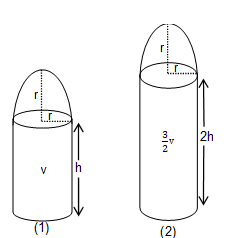Let v’ = increased volume when radii of hemisphere and cylinder are doubled,

Now,

v’ = π (2r)2h + (2/3)π(2r)3

v’ = 4 π r2h + (16/3)π r3

v’ = 4 ×(v/2) + 8 ×v/2 (from eq. (3))

v’ = 2v + 4v

v’= 6v

% change = (Δv/v) ×100

= ((vfinal - vinitial)/vinitial) ×100

= ((6v-v)/v) ×100

= 500%

Question 8: Consider a triangle PQR in which the relation QR2 + PR2 = 5 PQ2 hold. Let G be the point of intersection of medians PM and QN. Then ∠ QGM is always

1. a. Less than 450
2. b. obtuse
3. c. A right angle
4. d. Acute and larger than 450

Solution:

Let PQ = r

QR = p

RP = q

GM = (1/3)PM

QG = (2/3)QN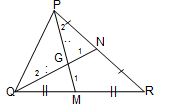Now,

QG2 + GM2 = (4/9)(QN)2 + (1/9)(PM)2

= (4(QN)2+(PM)2)/9 (using formula of length of median l= (1/2) √(2b2+2c2-a2 )

{QN = (1/2) √(2r2+2p2-q2 ); PM = (1/2) √(2r2+2q2-p2 )}

= (1/9)[4×(1/4)( 2r2+2p2-q2)+(1/4)( 2r2+2p2-p2)]

= (1/9)[2r2+2p2-q2+(1/4)(2r2+2q2-p2)]

= (1/36)[8r2+8p2-4q2+2r2+2q2-p2]

= (1/36)[10r2+7p2-2q2 ]

= (1/36)[2p2+2q2+7p2 -2q2 ] {Since QR2+PR2= 5PQ2; P2+q2 = 5r2}

= p2/4

= QM2

Hence angle QGM = 900 (using property of right angle triangle)

Question 9: Let a,b,c be the side-lengths of a triangle, and l, m, n be the lengths of its medians. Let K = (l+m+n)/(a+b+c). Then as a, b, c vary, K can assume every value in the interval

1. a. (1/4, 2/3)
2. b. (1/2, 4/5)
3. c. (3/4, 1)
4. d. (4/5, 5/4)

Solution:

l < (b+c)/2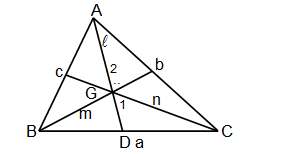Similarly m< (c+a)/2 and n < (a+b)/2

l+ m + n < a + b + c

(l+m+n)/(a+b+c) < 1 …….(1)

Also in the ΔBGC

BG + GC> BC
⇒ (2/3)(m+n) >a

Similarly (2/3)(n+l)>b and (2/3)(l+m) >c

Hence, (4/3)(l+m+n)>a+b+c

⇒ (l+m+n)/(a+b+c) > 3/4……..(2)

From eq. (1) and (2)

(l+m+n)/(a+b+c) ∈(3/4,1).

Question 10: Let x0, y0 be fixed real numbers such that x02+y02 >1. If x,y are arbitrary real numbers such that x2 + y2 ≤ 1, then the minimum value of (x – x0)2 + (y – y0)2 is

1. a. (√(x02+y02 )-1)2
2. b. x02+y02-1
3. c. (|x02 |+ |y02 |-1)2
4. d. (|x02|+ |y0 |)2-1

Solution:

Let circle x2 + y2 = 1 ;

C : (0, 0) and r = 1

⇒ x02+y02 >1 (given)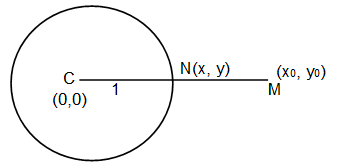So point (x0,y0) lies outside the circle x2 + y2 = 1

{using concept of position of point with respect to circle}

And x2+y2 ≤1 (given)

Hence point (x, y) lies inside or on the circle

{Using concept of position of point with respect to circle}

Now,

(x-x0)2+(y-y0)2 will be minimum when the variable point (x, y) will be on circle

min ={ ((x-x0)2+(y-y0)2 )}= (CM-CN)2

= [√(x02 +y02)-1)]2

{use d = √((x1-x2)2+(y1-y2)2 )}

Question 11: Let PQR be a triangle in which PQ = 3. From the vertex R, draw the altitude RS to meet PQ at S. Assume that RS = √3 and PS = QR. Then PR equals

1. a. √5
2. b. √6
3. c. √7
4. d. √8

Solution:

In Δ SRQ

Using Pythagoras theorem (RQ)2 = (RS)2+(SQ)2

⇒ x2 = (√3)2+ (3-x)2

⇒ x2 = 3 + 9 + x2 – 6x

⇒ 6x = 12

⇒ x = 2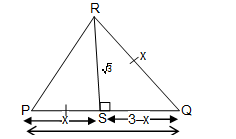Now,

In Δ PSR

Using Pythagoras theorem (PR)2 = (PS)2 + (RS)2

⇒ x2 + (√3)2 = (PR)2 (since x = 2)

⇒ 4 + 3 = (PR)2

⇒ PR = √7

Question 12: A 100 mark examination was administered to a class of 50 students. Despite only integer marks being given, the average score of the class was 47.5. Then, the maximum number of students who would get marks more than the class average is

1. a. 25
2. b. 35
3. c. 45
4. d. 49

Solution:

Total students in class = 50

Average score of class = 47.5

Total score of class = 50 × 47.5 = 2375

Student can obtain only integer marks. If 48 marks are obtained by student then maximum number of student can be = [2375/48]

= 49

Here [•] is greatest integer function.

Question 13: Let s be the sum of the digits of the number 152 × 518 in base 10. Then

1. a. s < 6
2. b. 6 ≤ s < 140
3. c. 140 ≤ s < 148
4. d. S ≥148

Solution:

N = 152 × 518

N = 32 × 520

= 32 × (10/2)20

On taking log base 10 both sides

log10N = log1032(10/2)20

= 2log103+20 log1010-20 log102{using log properties}

= 2 × .4771 + 20 (1 – .3010) = 14.64 {log10 3 = 0.4771; log10 2 = 0.3010}

Hence number of digit are 14 + 1 = 15

If each digit are 9 then maximum sum = 15 × 9 = 135 and

If number has only 5 and 3 as factor

So number would end with 5 hence sum of digits would be atleast 5, also as 3 is a factor so sum of digits should be divisible by 3

Hence, 6 would be minimum sum of digits

⇒ 6 ≤ S ≤135

From option 6 ≤ S < 140.

Question 14: Let PQR be an acute-angled triangle in which PQ < QR. From the vertex Q draw the altitude Q Q1, the angle bisector Q Q2 and the medium Q Q3 with Q1, Q2, Q3 lying on PR. Then.

1. a. PQ1< PQ2< PQ3
2. b. PQ2< PQ1< PQ3
3. c. PQ1< PQ3< PQ2
4. d. PQ3< PQ1< PQ2

Solution:

PQ3 = Q3R (since QQ3 is median)

PQ3 = ½ PR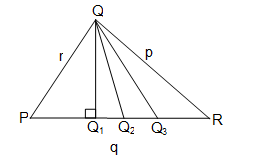PQ2 : Q2 R = r : p {By property of angle bisector}

Q2R/PQ2 = p/r

Apply componendo

(PQ2+Q2R)/PQ2 = (p+r)/r

PQ2 =(r/r+p) PR since {PQ2 + Q2R = PR}

But r < p (given)

Hence PQ2< ½ PR

Comparison between altitude and angle bisector

∠ QPQ2+ ∠ PQ2Q + ∠ PQQ2 = ∠ RQQ2 + ∠ QQ2R + ∠ QRQ2

Therefore ∠ PQQ2 = ∠RQQ2 {Since angle bisector}

∠ QPQ2 + ∠ PQ2Q = ∠ QQ2R + ∠ QRQ2

Therefore PQ < QR then ∠ QPQ2> ∠ QRQ2

Hence ∠ QQ2P < ∠ QQ2R

But ∠ QQ2P + ∠ QQ2R = 1800

Hence ∠ QQ2P < 900 and ∠ QQ2R > 900

⇒ foot from Q to side PR lies inside ΔPQQ2

⇒ PQ1< PQ2< PQ3

Question 15: All the vertices of rectangle are of the form (a, b) with a, b integers satisfying the equation (a–8)2–(b–7)2 = 5. Then the perimeter of the rectangle is

1. a. 20
2. b. 22
3. c. 24
4. d. 26

Solution:

(a –8)2 – (b – 7)2 = 5 (given)

Let a – 8 = x and b –7 = y

⇒ x2 – y2 = 5 {using A2 – B2 = (A – B) (A + B); where A = x, B = y

⇒ (x – y) (x + y) = 5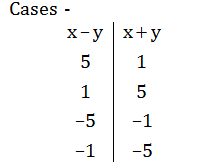Case I

x – y = 5 and x + y = 1

on solving

x = 3 and y = –2 (since a – 8 = x; b – 7 = y)

⇒ a – 8 = 3 and b–7 = –2

⇒ a = 11 and b = 5

Case II

x – y = 1 and x + y = 5

⇒ x = 3 and y = 2 {since a – 8 = x; b – 7 = y}

⇒ a = 11 and b = 9

Case III

x – y = –5 and x + y = –1

⇒ x = – 3 and y = 2 {since a – 8 = x; b – 7 = y}

⇒ a = 5 and b = 9

Case IV

x – y = –1 and x + y = –5

⇒ x = –3 and y = –2 { since a – 8 = x; b – 7 = y}

⇒ a = 5 and b = 5

Points of rectangle are (11, 5), (11, 9), (5, 5), (5, 9)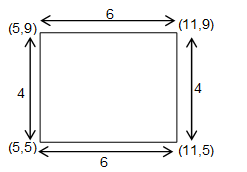Perimeter = 4 + 4 + 6 + 6 = 20

Question 16: What is the sum of all natural number n such that the product of the digits of n (in base 10) is equal to n2–10n–36?

1. a. 12
2. b. 13
3. c. 124
4. d. 2612

Solution:

Let product of digits of n be P(n)

Let n = ak10k + ak–110k–1 + ………..+ a0

P(n) = ak • ak–1 • ……………a1 • a0

Also

a0, a1 …………..ak–1 can take values from 0 to 9

therefore (a0 • a1 …………ak–1)max = 9k { a0 = a1 = a2 = ….ak–1 = 9}

hence P(n) ≤ ak 9k

P(n) ≤ ak(10)k ………(1)

Also

Ak10k ≤ ak10k + ak–110k–1+ ………+ a0 ≤ n …….(2)

From (1) and (2)

P(n) ≤ n

⇒ n2 –10n – 36≤ n

⇒ –2.64 ≤ n ≤ 13.64 ………(3)

Also P(n) ≥ 0

⇒ n2 – 10n–36≥ 0

⇒ n≥ 12.81 ….….(4)

From (3) and (4)

12.81 ≤ n ≤ 13.64

Hence n = 13 (since n belongs to I).

Question 17: Let m (respectively, n) be the number of 5-digit integers obtained by using the digits 1,2,3,4,5 with repetitions (respectively, without repetitions) such that the sum of any two adjacent digits is odd. Then m/n is equal to

1. a. 9
2. b. 12
3. c. 15
4. d. 18

Solution:

Case I

For (n); Repetition not allowed

⇒ OEOEO is the only possibility of arrangement of digits, where 0 = odd digit, E = even digits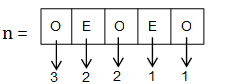n = 3 × 2 × 2 × 1 × 1 = 12

Case II

For (m) ; Repetition is allowed

⇒ two possibilities

(a) OEOEO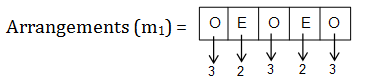m1 = 3 × 2 × 3 × 2 × 3 = 108

(b) EOEOE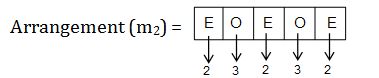m2 = 2 × 3 × 2 × 3 × 2 = 72

m = m1 + m2

m = 108 + 72 = 180

m/n = 180/12 = 15

Question 18: The number of solid cones with integer radius and height each having its volume numerically equal to its total surface area is

1. a. 0
2. b. 1
3. c. 2
4. d. infinite

Solution:

Let height = h, radius = r & slant height = l

Given, Volume = surface area

⇒ (1/3) πr2h = πrl + πr2 [since l = √(r2+h2) ]

⇒ rh = 3√(r2+h2) +3r

⇒ rh – 3r = 3√(r2+h2)

On squaring both sides

⇒ (rh – 3r)2 = (3√(r2+h2))2 {using (A – B)2 = A2 + B2– 2AB}

⇒ r2h2 + 9r2 – 6r2h = 9r2 + 9h2

⇒ r2(h – 6) = 9h

⇒ r2 = 9h/(h-6)

⇒ r2 = (9(h-6)+54)/(h-6)

= 9 + (54/(h-6)

There exists only one solution which satisfy above condition is h = 8, and r = 6.

Question 19: Let ABCD be a square. An arc of a circle with A as center and AB as radius is drawn inside the square joining the points B and D. Points P on AB, S on AD, Q and R on arc BD are taken such that PQRS is a square. Further suppose that PQ and RS are parallel to AC. Then area PQRS/area ABCD is

1. a. 1/8
2. b. 1/5
3. c. ¼
4. d. 2/5

Solution:

Let, A(0, 0), B (x1, 0), C(x1, x1) and D(0, x1)

Area of ABCD = (x1)2 {since area of square = (side)2}

Let P(a, 0) and S(0, b)

Equation of circle is x2 + y2 = x12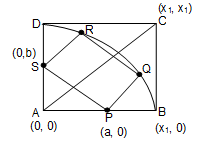Equation of circle is x2 + y2 = x12

Slope of AC = (x1-0)/ (x1-0) = 1 {since slope = (y2-y1)/(x2-x1) }

Slope of PQ and SR = 1

⇒ θ = π/4

Let PQ = SR = d

Using parametric coordinate (x1 ±r cos θ, y1 ±r sin θ)

Q = (a + dcos450, 0 + dsin450) = (a+d/√2, d/√2) { Here (x1, y1) = (a, 0); r = d; θ = π /4}

R = (0 + dcos450; b + dsin450) = (d/√2, b+d/√2) { Here (x1, y1) = (0, b); r = d; θ = π /4 }

Q and R lies on Arc BD

⇒ (a+d/√2)2+(d/√2)2 = x12

⇒ a2 + d2 +√2ad = x12 ………..(1)

Similarly b2 + d2 +√2bd = x12 ……….(2)

And

(PS)2 = (d)2

⇒ a2 + b2 = d2 ……….(3)

⇒ Slope of PS = –1 (PS perpendicular AC) {since slope of AC = 1}

⇒ -b/a = –1

⇒ a = b ……………(4)

From (3) and (4)

⇒ 2a2 = d2

⇒ a = d/√2 = b put in eq (1)

Now (d2/2)+ d2 + d2 = x12

⇒ d2 = (2/5) x12

= Area (PQRS)

⇒ area PQRS/area ABCD = (2/5)x12/x12

= 2/5

Question 20: Suppose ABCD is a trapezium whose sides and height are integers and AB is parallel to CD. If the area of ABCD is 12 and the sides are distinct, then |AB – CD|

1. a. is 2
2. b. is 4
3. c. is 8
4. d. cannot be determined from the data

Solution:

Area = 12

⇒ (1/2)(a + b) × h = 12

⇒ (a + b) × h = 24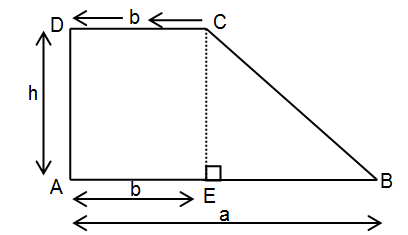Possible cases-> 24 × 1

12 ×2

6×4

8×3

In ΔEBC, possible height for integer sides, h = 4, 3

Case I When h = 4

Then possible triplet (3, 4, 5)

i.e. EC = 4, EB = 3, BC = 5

it EB = 3, 2b = 3

b = 3/2 (not possible because b belongs to I)

Case II When h = 3

Then EB = 4

2b = 4

b = 2

Hence CD = 2, AB = 6

So |AB – CD| = 4

### KVPY-SA 2018 Maths Paper with Solutions